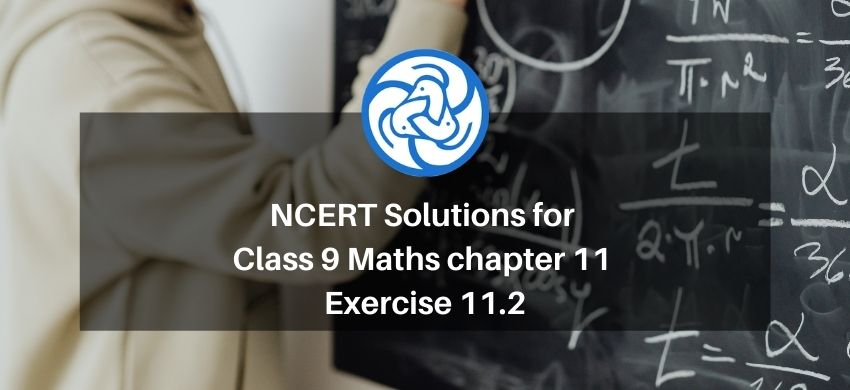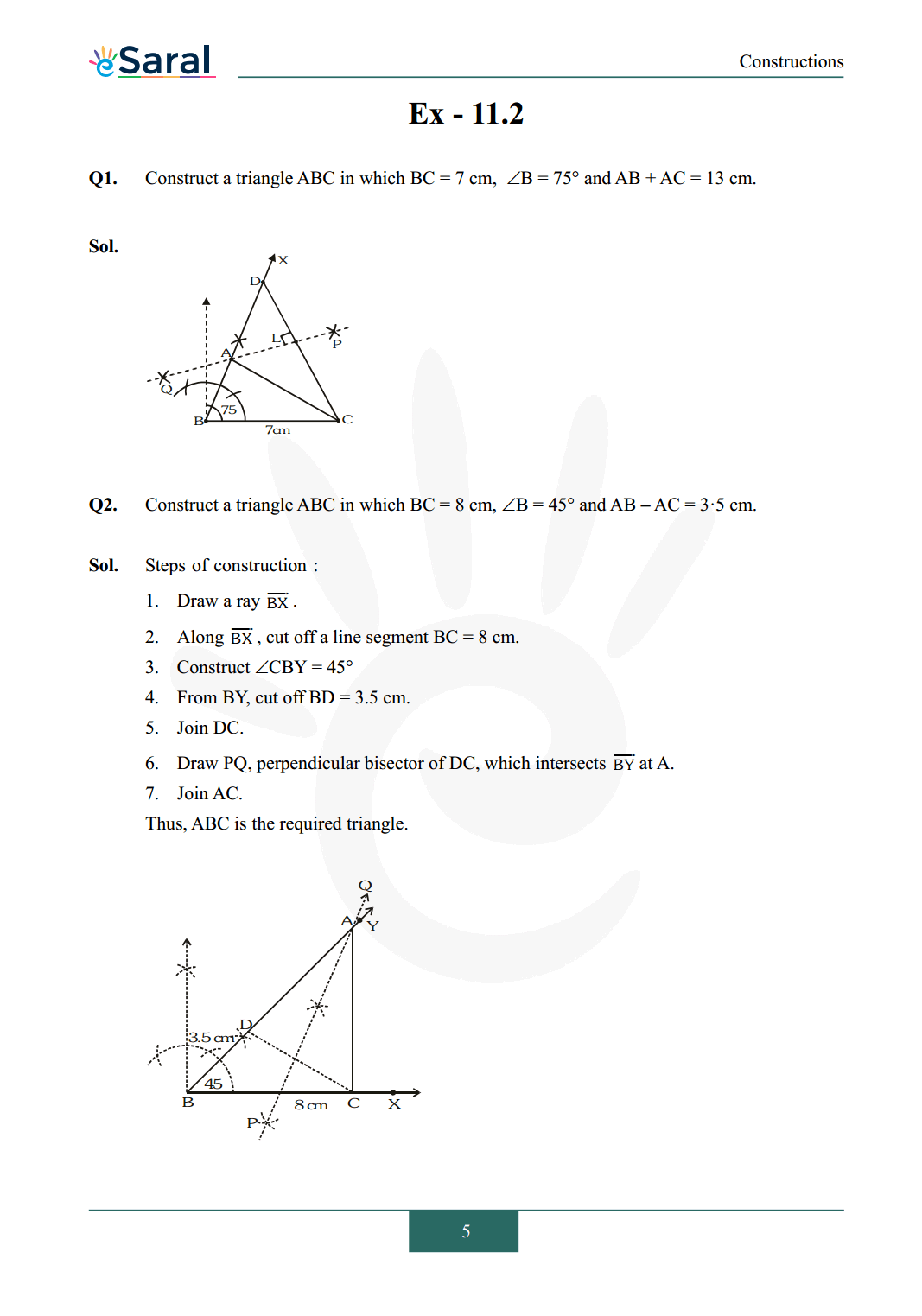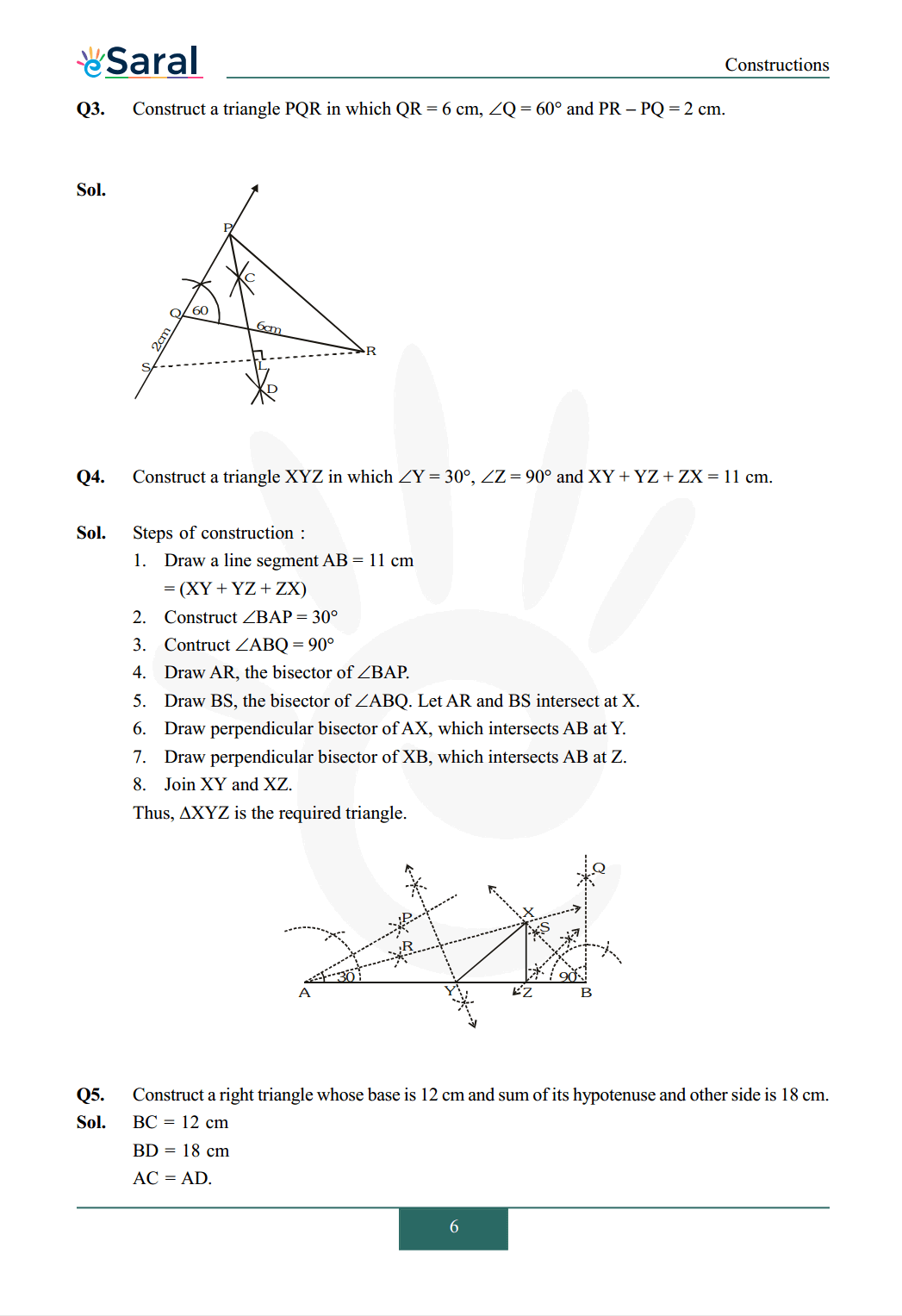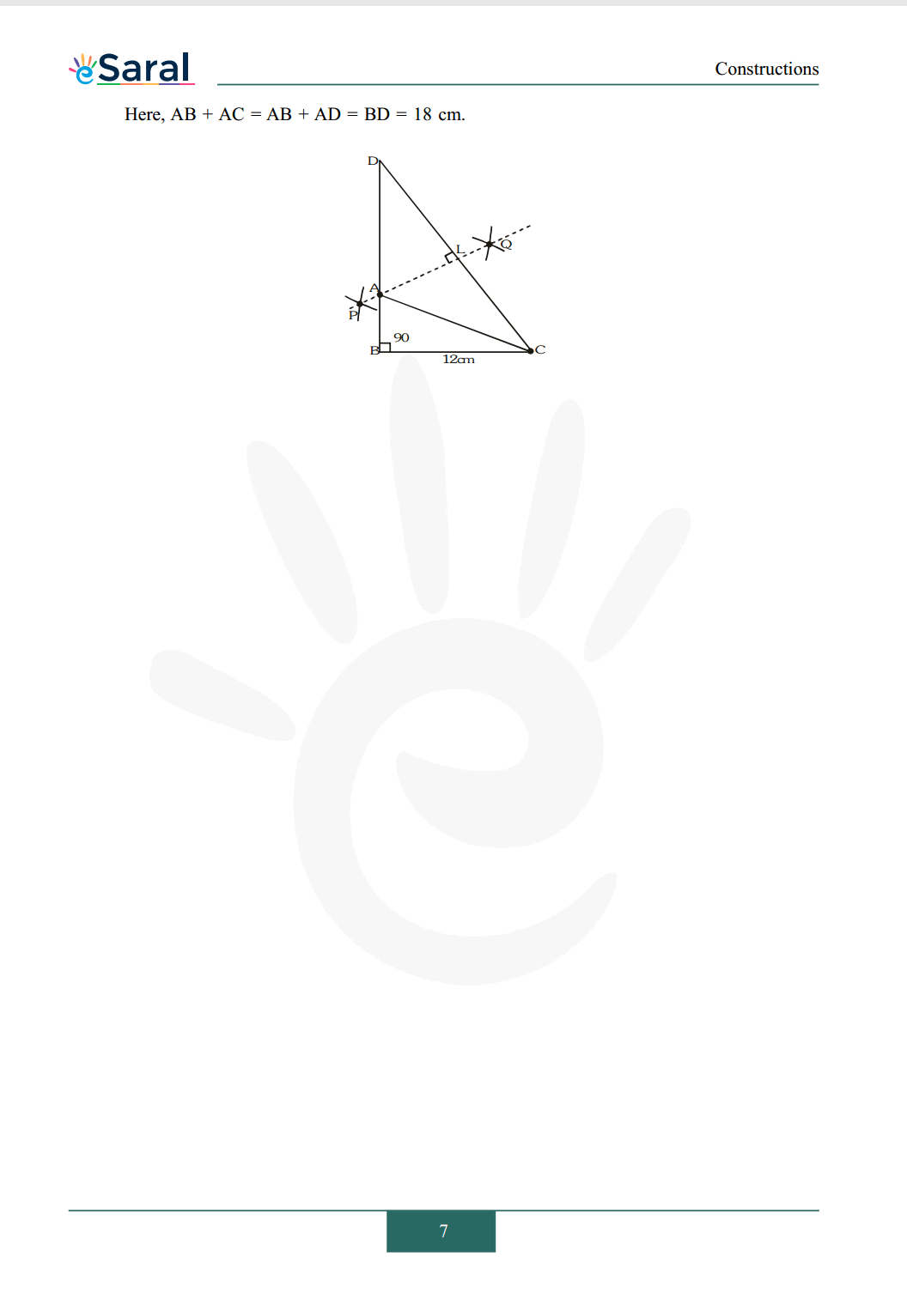Most Affordable JEE | NEET | 8,9,10 Preparation by Kota's Top IITian Doctor Faculties

# NCERT Solutions for Class 9 Maths chapter 11 Exercise 11.2 - Constructions`
Hey, are you a class 9 Student and Looking for Ways to Download NCERT Solutions for Class 9 Maths chapter 11 Exercise 11.2? If Yes then you are at the right place.

Here we have listed Class 9 maths chapter 11 exercise 11.2 solutions in PDF that is prepared by Kota’s top IITian’s Faculties by keeping Simplicity in mind.

If you want to score high in your class 9 Maths Exam then it is very important for you to have a good knowledge of all the important topics, so to learn and practice those topics you can use eSaral NCERT Solutions.

So, without wasting more time Let’s start.

### Download The PDF of NCERT Solutions for Class 9 Maths chapter 11 Exercise 11.2 "Constructions"#### All Questions of Chapter 11 Exercise 11.2

Once you complete the chapter 11 then you can revise Ex. 11.2 by solving following questions

Q1. Construct a triangle ABC in which BC = 7 cm, B = 75° and AB + AC = 13 cm.

Q2. $\quad$ Construct a triangle $\mathrm{ABC}$ in which $\mathrm{BC}=8 \mathrm{~cm}, \angle \mathrm{B}=45^{\circ}$ and $\mathrm{AB}-\mathrm{AC}=3 \cdot 5 \mathrm{~cm}$.

Q3. Construct a triangle $\mathrm{PQR}$ in which $\mathrm{QR}=6 \mathrm{~cm}, \angle \mathrm{Q}=60^{\circ}$ and $\mathrm{PR}-\mathrm{PQ}=2 \mathrm{~cm}$.

Q4. Construct a triangle $\mathrm{XYZ}$ in which $\angle \mathrm{Y}=30^{\circ}, \angle \mathrm{Z}=90^{\circ}$ and $\mathrm{XY}+\mathrm{YZ}+\mathrm{ZX}=11 \mathrm{~cm}$.

Q5. Construct a right triangle whose base is 12 cm and sum of its hypotenuse and other side is 18 cm.